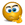# How to test Statistical significance of portfolio in a spreadsheet.

## Excel Help for How To Test Statistical Significance Of Portfolio In A Spreadsheet. in Managing and Analyzing Financial Portfolios

### How To Test Statistical Significance Of Portfolio In A Spreadsheet.

Rate this:
(3/5 from 1 vote)Hi,Assume that i track 10 different portfolios with 10 stocks in single portfolio, for 15 trading days. So how can I determine which portfolio returns are best with low risk profile and high returns in an excel spreadsheet.In respect to statistical proven results and formulas.Waiting for ur reply.Regards,Vijay Posted by vijay_06 on 13 May 2006
 Replies - Displaying 1 to 3 of 3 Order Replies By: Most Recent | Chronological | Highest RatedRate this:
(3/5 from 1 vote)
To evaluate the risk/return profiles of multiple portfolios within the same spreadsheet, you could create a worksheet for each portfolio and then an analysis sheet linking the results of each portfolio sheet for analysis.

For simplicity, suppose there were 3 stocks in each portfolio with 4 trading days.  Each portfolio sheet could be constructed as follows.

 A B C D E F G 1 2 No. Shares 20 30 25 3 Price data Portfolio Return Calc 4 Day 1 2 7 3 =SUMPRODUCT(\$B\$2:\$D\$2,B4:D4) =STDEV(F5:F7) 5 Day 2 3 9 2 =SUMPRODUCT(\$B\$2:\$D\$2,B5:D5) =(E5-E4)/E4 =AVERAGE(F5:F7) 6 Day 3 3 4 5 =SUMPRODUCT(\$B\$2:\$D\$2,B6:D6) =(E6-E5)/E5 0.01% 7 Day 4 4 5 7 =SUMPRODUCT(\$B\$2:\$D\$2,B7:D7) =(E7-E6)/E6 =(G5-G6)/G4

Columns A to D list the price data and number of shares held.  Column E calculates the total portfolio value for each day by using the SUMPRODUCT formula.  The formula in cell E4 can be copied or dragged down from the bottom right corner of the cell. Column F calculated the daily returns of the total portfolio value.

The performance calculation are made in column G. Cell G4 calculates the standard deviation of returns (risk).  Cell G5 calculates the average daily return.  The 1 day risk-free rate can be entered into cell G6.  Finally the Sharpe Ratio is calculated in cell G7 which summarizes the return/risk profile.  The portfolio with the highest Sharpe Ratio will have the best profile.

None of this analysis requires tests for statistical significance. If, however, you wanted to calculate the probability of achieving a certain return, risk or Sharpe Ratio for any portfolio then tests for statistical significance at a prescribed confidence interval would be recommended.Rate this: (3/5 from 1 vote) I can just say a big "Thanks" for providing informative stuff. accountant milton keynes Posted by accountant milton keynes on 18 Nov 2009Rate this: (3/5 from 1 vote) very informative thanks for sharing [url="http://www.1stclaims.co.UK/"]UK personal injury[/url] Posted by decklinbrain on 18 Dec 2009